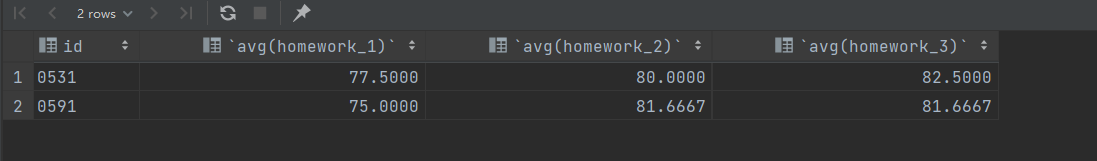# MySQL小练习（仅适合初学者，非初学者勿进）

时间：2022-05-11 15:09:10  阅读量：26  评论数：0  作者：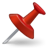个人主页：个人主页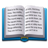​ 系列专栏：MySQL数据库

# 题目：

1. 查询各位学生的学号、班级和姓名
2. 查询课程的全部信息
3. 查询数据库中有哪些专业班级
4. 查询学时大于60的课程信息
5. 查询出生在1986年出生的学生的学号、姓名和出生日期
6. 查询三次作业成绩都在80分以上的学号、课程号
7. 查询姓张的学生的学号、姓名和专业班级
8. 查询05级的男生信息
9. 查询没有作业成绩的学号和课程号

10. 查询学号为0538的学生的作业1总分

11. 查询选修了K001课程的学生人数

12. 查询数据库中共有多少个班级

13. 查询选修三门以上（含3门)课程的学生的学号和作业1平均分，作业2平均分，作业3 平均分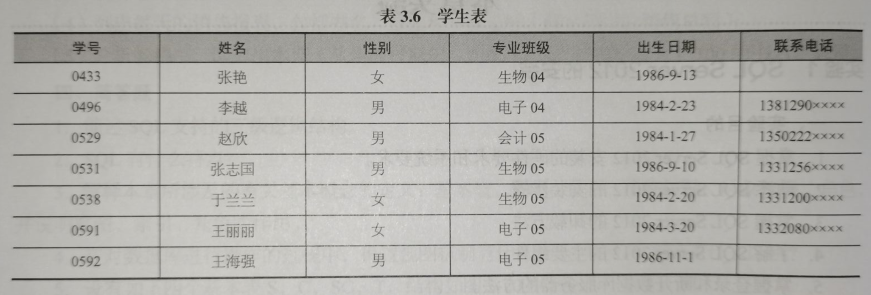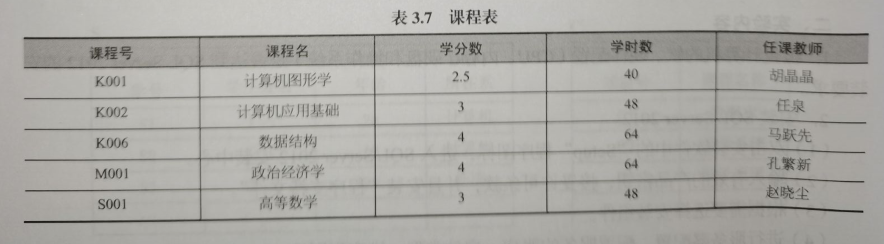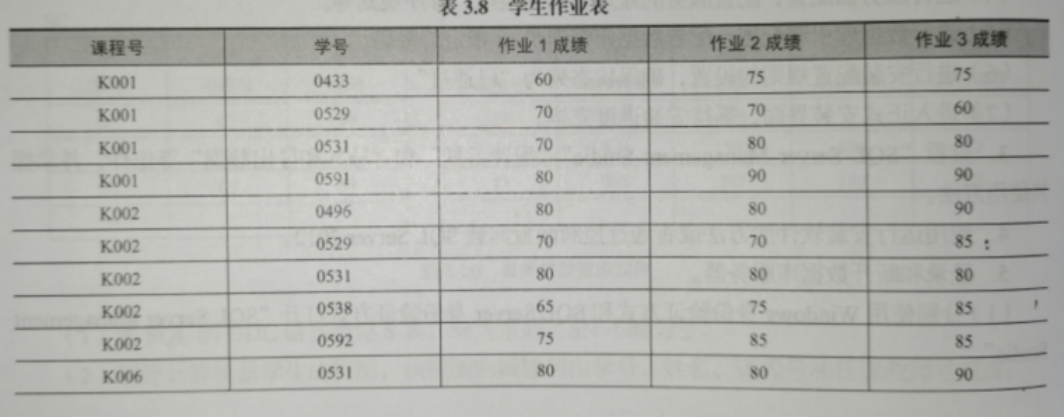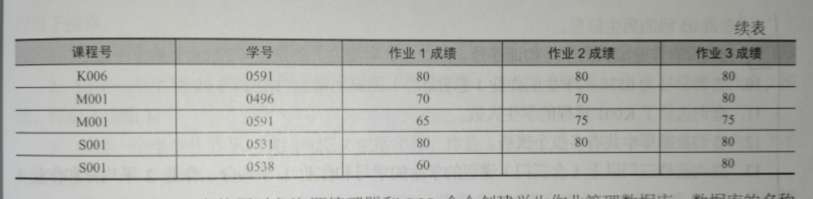2022最新MySQL基础（奋笔疾书4w字 只为博君看两眼）_一个热爱编程的小白白的博客-CSDN博客

# 1.创建数据库

``create database  if not exists  db2 ;``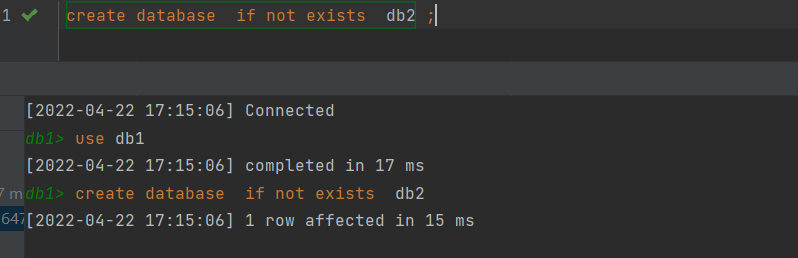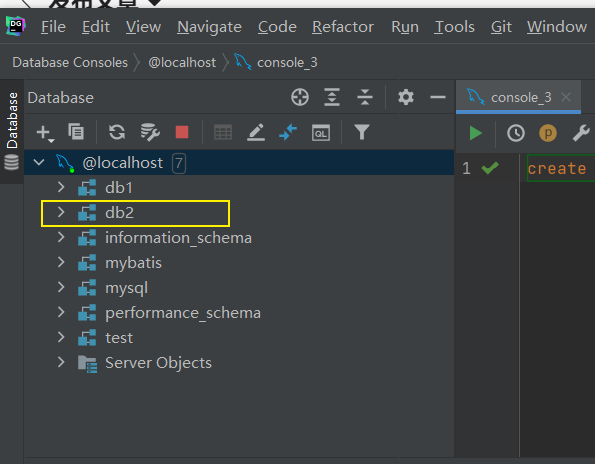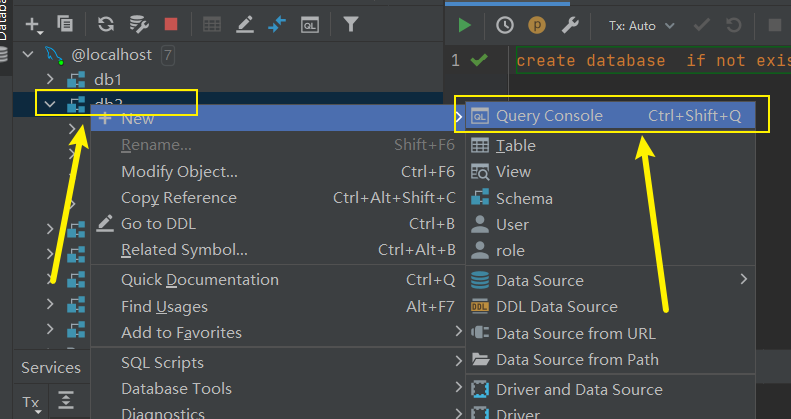# 2.创建表

## 1.创建学生表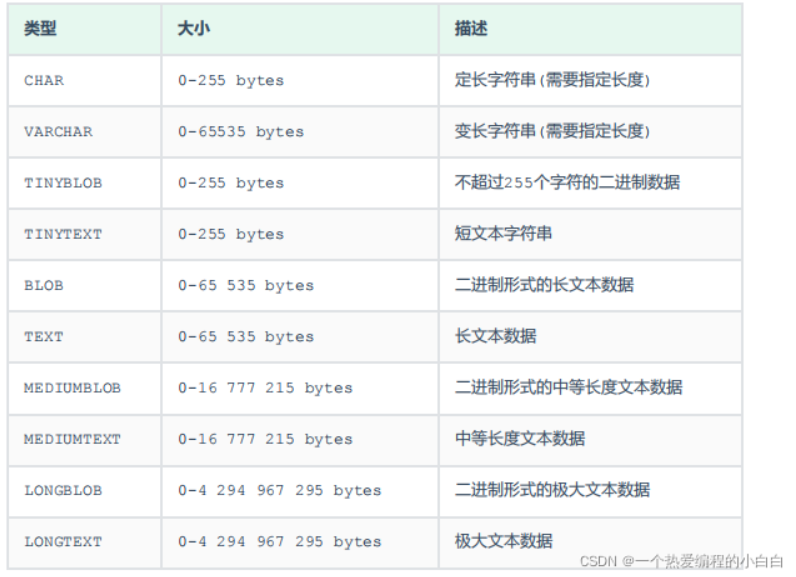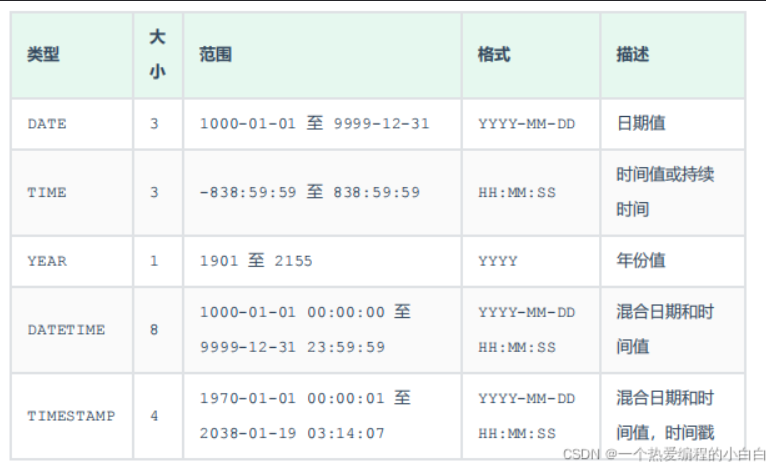``````drop table if exists student;
create table student
(
id     varchar(10) comment '学号',
name   varchar(10) NOT NULL comment '姓名',
gender char(1) comment '性别',
class  varchar(20) comment '专业班级',
date   date comment '出生日期',
iphone varchar(11) comment '联系电话'
)
comment '学生表';

select * from student;``````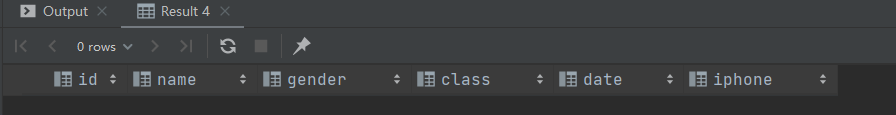## 2.创建课程表``````drop table if exists student_course;
create table student_course
(
course_id     varchar(10)  comment '课程号',
course_name   varchar(15) comment '课程名',
course_number double unsigned comment '学分数',
student_time  int unsigned comment '学时数',
teacher       varchar(10) comment '任课教师'
)
comment '课程表';
select *
from student_course;``````## 3.学生作业表``````drop table if exists student_homework;
create table student_homework
(
course_id  varchar(10) comment '课程号',
id      varchar(10)    comment '学号',
homework_1 int comment '作业1成绩',
homework_2 int comment '作业2成绩',
homework_3 int comment '作业3成绩'

)
comment '学生作业表';
select *
from student_homework;``````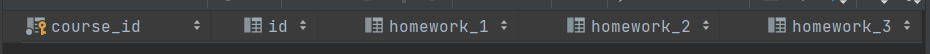# 3.添加数据

1.学生表``````insert into student
values ('0433', '张艳', '女', '生物04', '1986-9-13', null),
('0496', '李越', '男', '电子04', '1984-2-23', '1381290xxxx'),
('0529', '赵欣', '男', '会计05', '1984-1-27', '1350222xxxx'),
('0531', '张志国', '男', '生物05', '1986-9-10', '1331256xxxx'),
('0538', '于兰兰', '女', '生物05', '1984-2-20', '1331200xxxx'),
('0591', '王丽丽', '女', '电子05', '1984-3-20', '1332080xxxx'),
('0592', '王海强', '男', '电子05', '1986-11-1', null);``````

查询一下：

``select * from student;``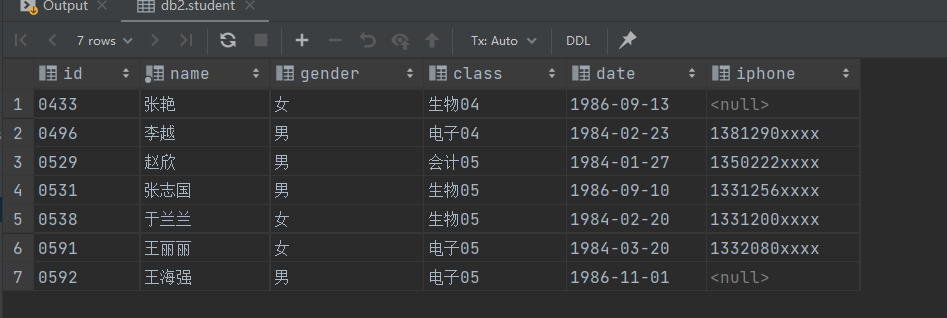2.课程表``````INSERT INTO student_course
values ('K001', '计算机图形学', 2.5, 40, '胡晶晶'),
('K002', '计算机应用基础', 3, 48, '任泉'),
('K006', '数据结构', 4, 64, '马跃先'),
('M001', '政治经济学', 4, 64, '孔繁新'),
('S001', '高等数学', 3, 48, '赵晓尘');``````

``````select *
from student_course;``````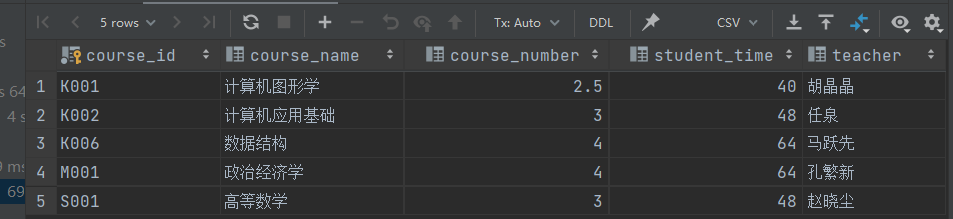3.学生作业表``````insert into student_homework values
('K001','0433',60,75,75),
('K001','0529',70,70,60),
('K001','0531',70,80,80),
('K001','0591',80,90,90),
('K002','0496',80,80,90),
('K002','0529',70,70,85),
('K002','0531',80,80,80),
('K002','0538',65,75,85),
('K002','0592',75,85,85),
('K006','0531',80,80,90),
('K006','0591',80,80,80),
('M001','0496',70,70,80),
('M001','0591',65,75,75),
('S001','0531',80,80,80),
('S001','0538',60,null,80);``````

``````select *
from student_homework;``````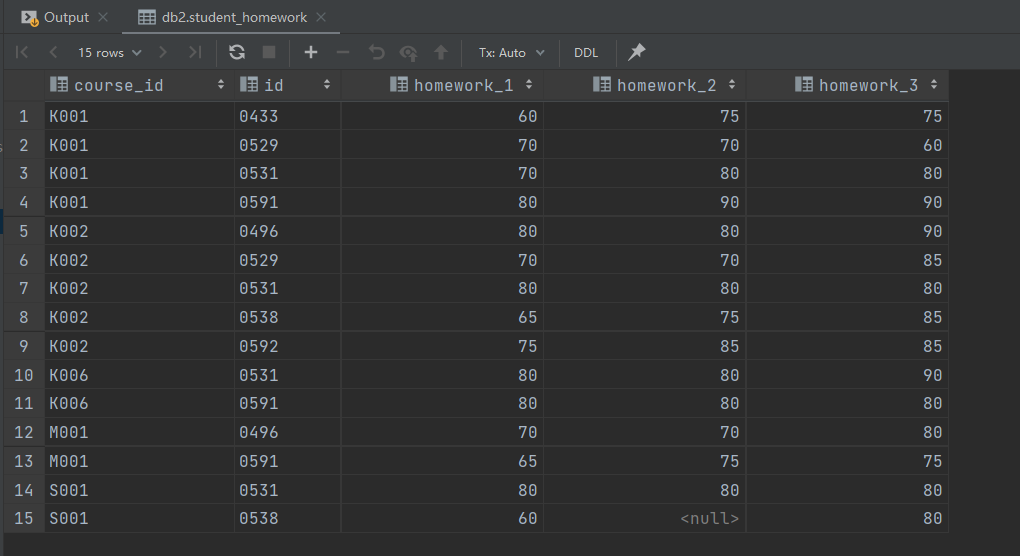# 4.开始做题

1.查询各位学生的学号、班级和姓名

``select id,class,name from student;``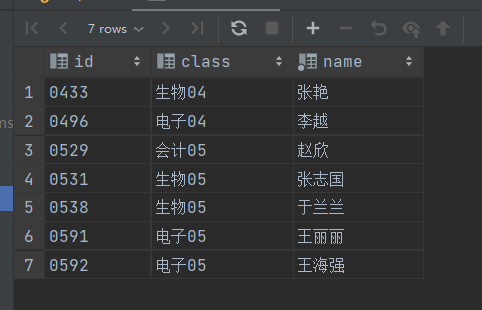2.查询课程的全部信息

``````select *
from student_course;``````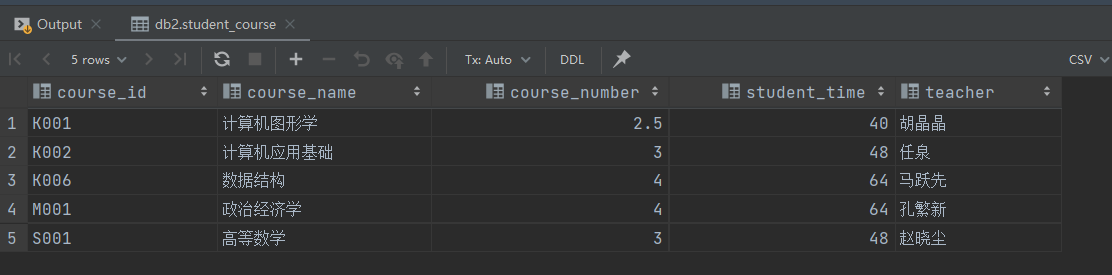3.查询数据库中有哪些专业班级

``select  class from student;``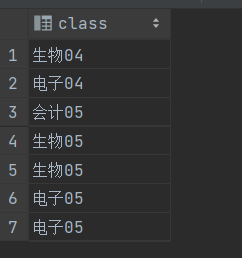4.查询学时大于60的课程信息

``select course_id,course_name from student_course where student_time>60;``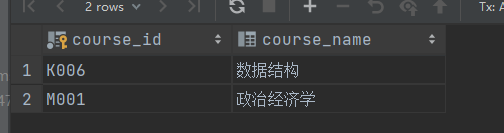5.查询出生在1986年出生的学生的学号、姓名和出生日期

``select id,name,date from student where date>=('1986-1-1') AND date<('1987-1-1');``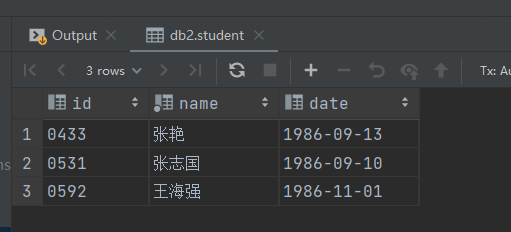6.查询三次作业成绩都在80分以上的学号、课程号

一开始我是用这个查询了一遍：

``select * from student_homework where homework_1>80 and homework_2>80 and homework_3>80;``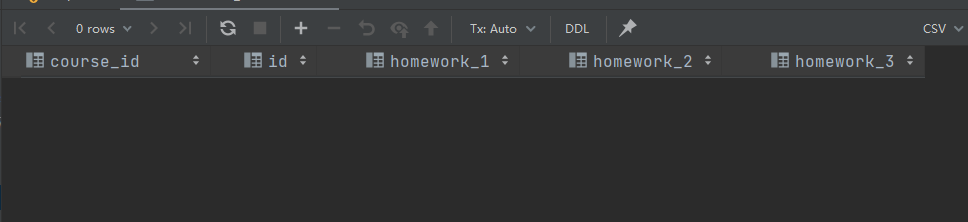``select * from student_homework where homework_1>=80 and homework_2>=80 and homework_3>=80;``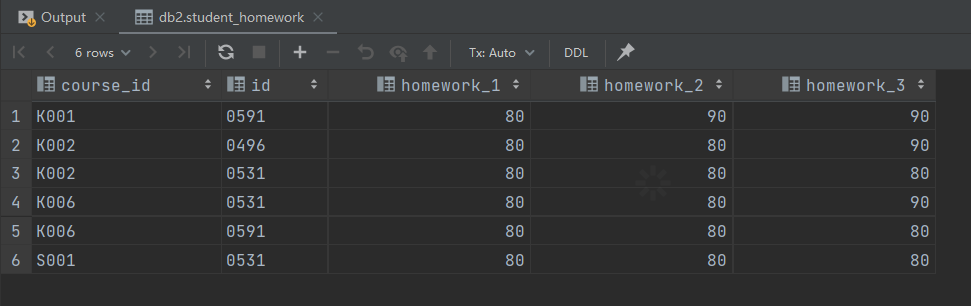7.查询姓张的学生的学号、姓名和专业班级

错误示范：

``select id,name,class from  student where name = '张%';``

``select id,name,class from  student where name like '张%';``

​​​​​​​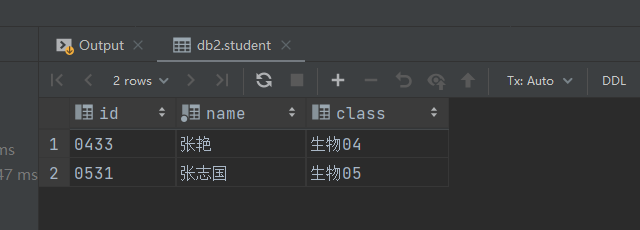8.查询05级的男生信息

``select * from student where class like '' and gender='男';``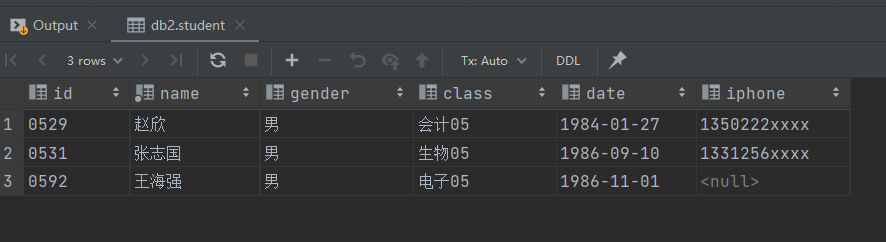9.查询没有作业成绩的学号和课程号

``select id,course_id from student_homework where homework_1 is null or homework_2 is null or homework_3 is null ;``

​​​​​​​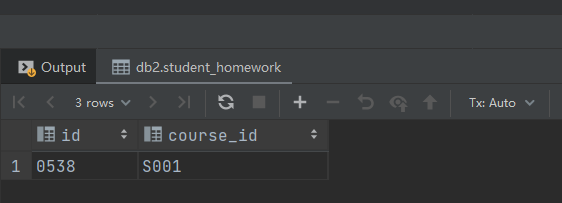10.查询学号为0538的学生的作业1总分

``select sum(homework_1) '总分' from student_homework where id='0538';``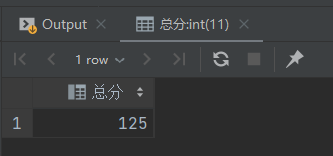11.查询选修了K001课程的学生人数

``select count(*) from student_homework where course_id='K001';``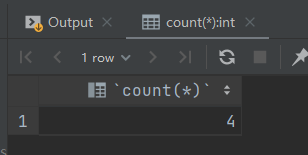12.查询数据库中共有多少个班级

``select count(*) from student where class is not null ;``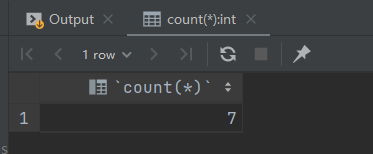13.查询选修三门以上（含3门)课程的学生的学号和作业1平均分，作业2平均分，作业3 平均分

``````select student.id, avg(homework_1), avg(homework_2), avg(homework_3)
from student
left join student_homework on student.id = student_homework.id
group by student.id
having count(course_id) >= 3;``````